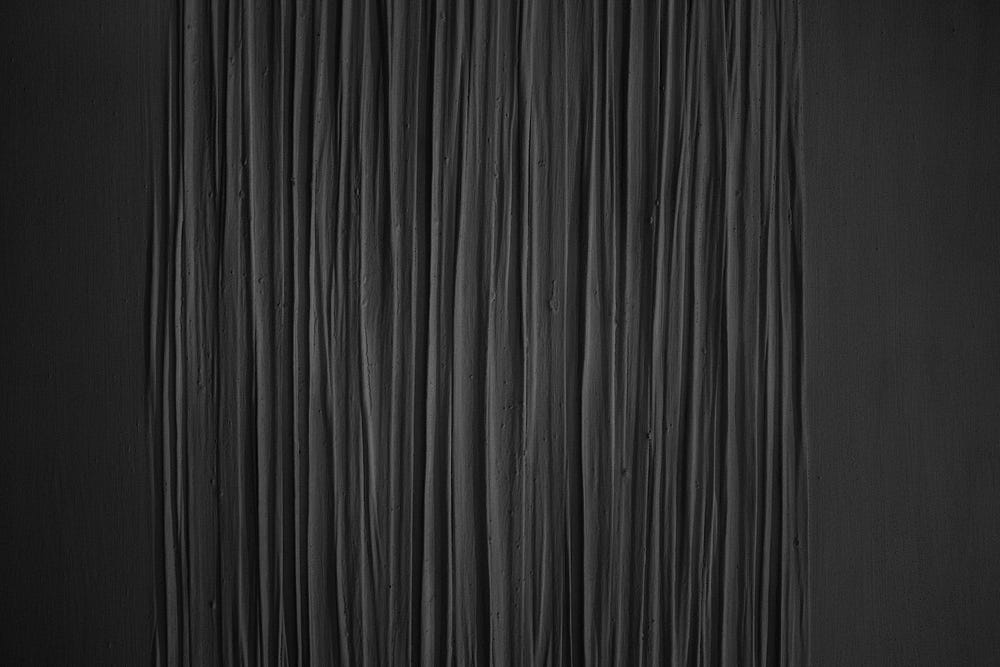# Cross entropy loss intuition

Source: Deep Learning on Medium# Cross entropy loss intuition

Given an input (and some classes), determine/predict the class to which the input belongs: this is a classification problem. Essentially, a model for probabilistic classification maps inputs to probabilistic predictions. For example, if we have 3 classes {(A)irplane, (B)oat, (C)ar}, our model might accept an image as a single observation input and return three numbers representing the probabilities of classes A, B and C as output. During training, we might feed an image of a car as input hoping that the model produces predictions that are close to the true (observed) class probabilities